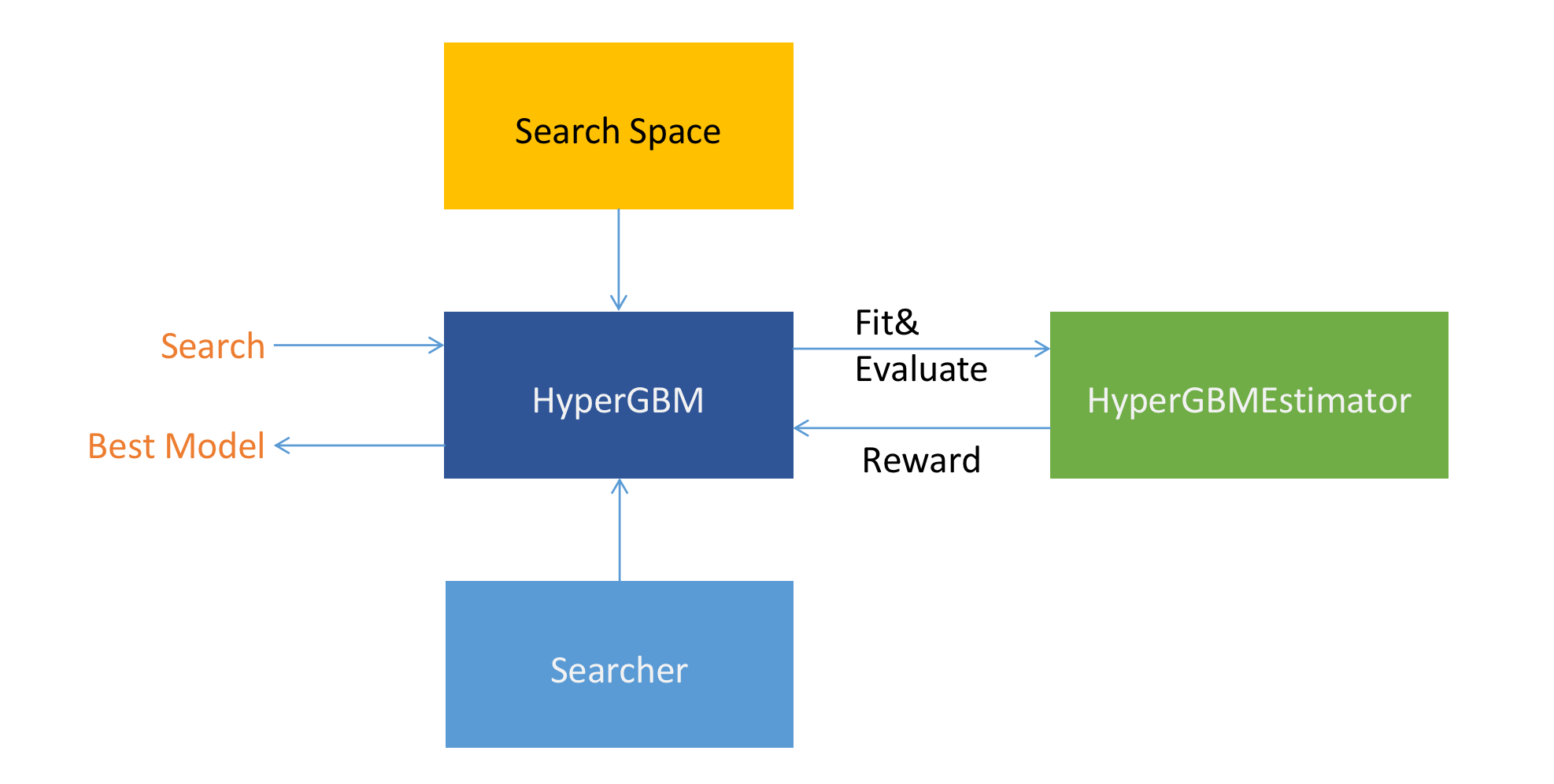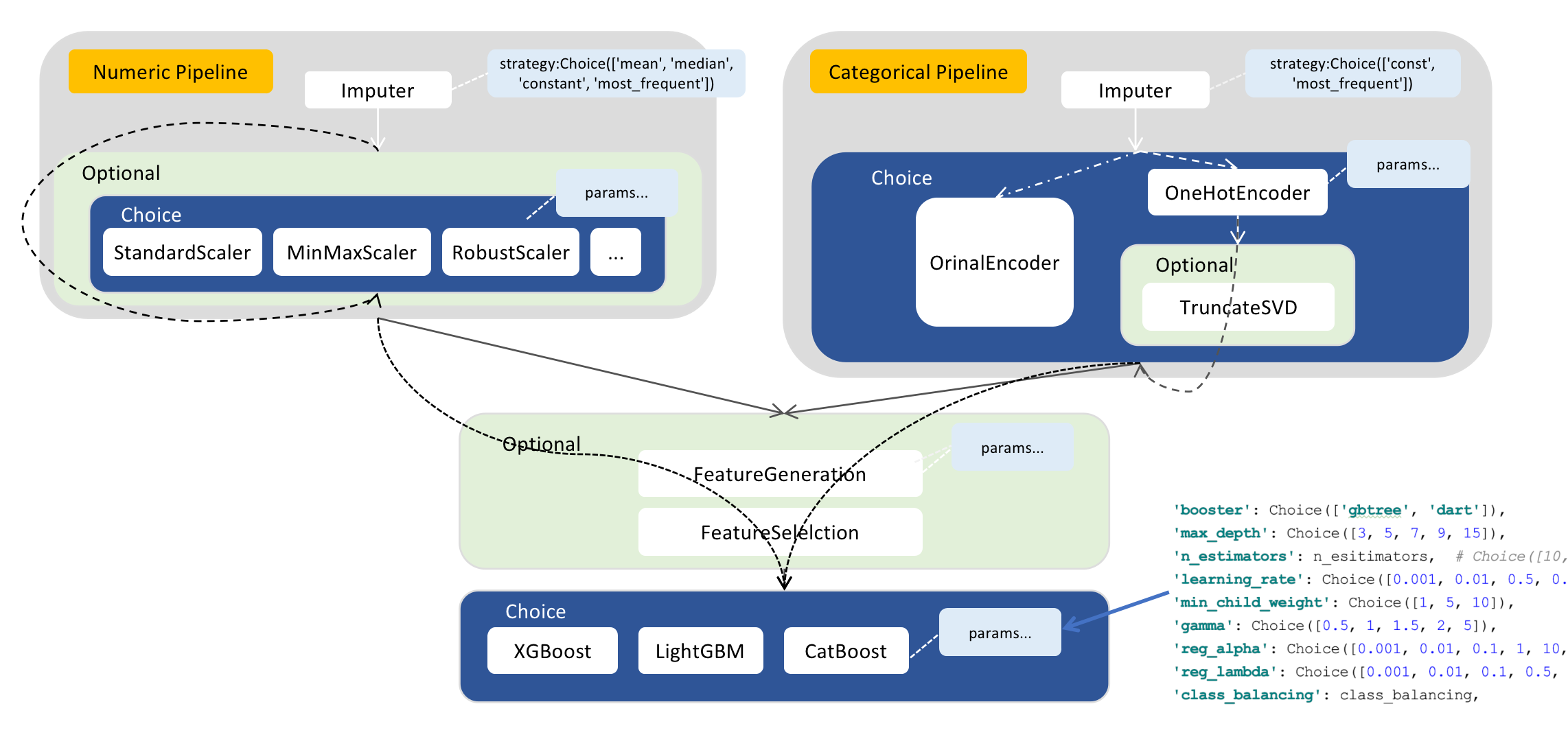# 概览¶

## HyperGBM是什么？¶

HyperGBM是一款全Pipeline自动机器学习工具，可以端到端的完整覆盖从数据清洗、预处理、特征加工和筛选以及模型选择和超参数优化的全过程，是一个真正的结构化数据AutoML工具包。

HyperGBM中的优化算法和搜索空间表示技术由 Hypernets 项目提供支撑。

## 核心组件¶• HyperGBM(HyperModel)

HyperGBM是HyperModel的一个具体实现（关于HyperModel的详细信息，请参考 Hypernets 。 HyperGBM类是该项目最主要的接口，可以通过它提供的 `search` 方法来完成使用特定的 `Searcher` (搜索器)在特定 `Search Space` (搜索空间)中搜索并返回最佳的模型，简单来说就是一次自动建模的过程。

• Search Space（搜索空间）

HyperGBM中的搜索空间由ModelSpace（各种Transformer和Estimator）,ConnectionSpace（Pipeline，实现Model间的连接和排列）,ParameterSpace（各种类型的超参数）三种搜索组件组合构成。Transformer使用Pipeline按顺序链接起来，Pipeline可以嵌套，这样可以实现非常复杂的多层次数据处理管道，整个搜索空间的最后一个节点只能是Estimator对象。所有的Transformer和Estimator都可以包含一组超参数搜索空间。 下图是一个搜索空间的示例：```import numpy as np
from hypergbm.pipeline import Pipeline
from hypergbm.sklearn.transformers import SimpleImputer, StandardScaler, MinMaxScaler, MaxAbsScaler, RobustScaler, LogStandardScaler
from hypernets.core.ops import ModuleChoice, Optional, Choice
from tabular_toolbox.column_selector import  column_number_exclude_timedelta

def numeric_pipeline_complex(impute_strategy=None, seq_no=0):
if impute_strategy is None:
impute_strategy = Choice(['mean', 'median', 'constant', 'most_frequent'])
elif isinstance(impute_strategy, list):
impute_strategy = Choice(impute_strategy)

imputer = SimpleImputer(missing_values=np.nan, strategy=impute_strategy, name=f'numeric_imputer_{seq_no}',
force_output_as_float=True)
scaler_options = ModuleChoice(
[
LogStandardScaler(name=f'numeric_log_standard_scaler_{seq_no}'),
StandardScaler(name=f'numeric_standard_scaler_{seq_no}'),
MinMaxScaler(name=f'numeric_minmax_scaler_{seq_no}'),
MaxAbsScaler(name=f'numeric_maxabs_scaler_{seq_no}'),
RobustScaler(name=f'numeric_robust_scaler_{seq_no}')
], name=f'numeric_or_scaler_{seq_no}'
)
pipeline = Pipeline([imputer, scaler_optional],
name=f'numeric_pipeline_complex_{seq_no}',
columns=column_number_exclude_timedelta)
return pipeline
```
• Searcher

Searcher是用于在搜索空间中完成搜索过程的算法。一个搜索算法最核心的部分就是如何平衡exploration（勘探）和exploitation（开采）的策略，一方面要能够快速的逼近全局最优解，另一方面要避免陷入局部某个次优的局部空间。 在HyperGBM中提供了MCTSSearcher（蒙特卡洛树搜索）、EvolutionarySearcher（进化搜索）和RandomSearcher（随机搜索）三种算法。

• HyperGBMEstimator

HyperGBMEstimator是根据搜索空间中的一个样本来构建的评估器对象，其中包括完整的预处理器管道（Preprocessing Pipeline）和 一个特定的GBM算法模型，可以用来在训练集上 `fit` ，用评估集来 `evaluate` 以及在新数据上完成 `predict`

• CompeteExperiment

`CompeteExperiment` 是HyperGBM提供的一个强大的工具，它不但可以完成pipeline搜索，同时还包括了一系列高级特性来进一步提升模型的性能，包括data drift handling（数据漂移处理）、pseudo-labeling（伪标签-半监督学习）、ensemble等等。

## 特性矩阵¶

• 单机模式

• 单机分布式

• 多机分布式

#

id列清理

XGBoost
Catboost
LightGBM
HistGridientBoosting

Train-Validation-Holdout验证

Class Weight

n次搜索都不再提升,提前停止

expected_reward